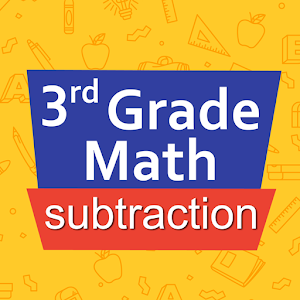Português

Todos os dias nós oferecemos aplicativos e jogos para Android licenciados GRATUITAMENTE que, caso contrário, você teria que comprar.\$2.99

# Android Giveaway of the Day - Third grade Math - Subtraction

An assistant and training suite for subtraction.
avaliação do usuário: 2 (100%) 0 (0%)

This giveaway offer has been expired. Third grade Math - Subtraction is now available on the regular basis.

Intuitive interface powered by handwriting input and a fun and engaging mini game in addition to a regular math trainer mode make our app stand out from the crowd of generic math learning apps.

With Third grade Math - Subtraction you can practice and improve the following math skills:
- Subtraction facts - numbers up to 100
- Subtract two two-digit numbers
- Subtract two three-digit numbers
- Complete the subtraction sentence up to three digits
- Subtract numbers with four or five digits
- Balance subtraction equations up to two digits
- Balance subtraction equations up to three digits

Sergey Malugin

Education

### Versão:

Varies with device

### Tamanho:

Varies with device

### Avaliação:

Everyone